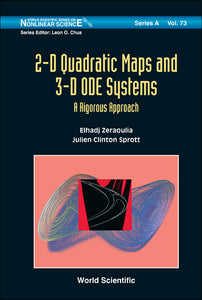# 2-d Quadratic Maps And 3-d Ode Systems: A Rigorous Approach

Format: Print Book

ISBN: 9789814307741

• SGD 186.18
Unit price per
Tax included.

This book is based on research on the rigorous proof of chaos and bifurcations in 2-D quadratic maps, especially the invertible case such as the Hénon map, and in 3-D ODE's, especially piecewise linear systems such as the Chua's circuit. In addition, the book covers some recent works in the field of general 2-D quadratic maps, especially their classification into equivalence classes, and finding regions for chaos, hyperchaos, and non-chaos in the space of bifurcation parameters.Following the main introduction to the rigorous tools used to prove chaos and bifurcations in the two representative systems, is the study of the invertible case of the 2-D quadratic map, where previous works are oriented toward Hénon mapping. 2-D quadratic maps are then classified into 30 maps with well-known formulas. Two proofs on the regions for chaos, hyperchaos, and non-chaos in the space of the bifurcation parameters are presented using a technique based on the second-derivative test and bounds for Lyapunov exponents. Also included is the proof of chaos in the piecewise linear Chua's system using two methods, the first of which is based on the construction of Poincaré map, and the second is based on a computer-assisted proof. Finally, a rigorous analysis is provided on the bifurcational phenomena in the piecewise linear Chua's system using both an analytical 2-D mapping and a 1-D approximated Poincaré mapping in addition to other analytical methods.

Format: Hardcover
No of Pages: 356
Imprint: World Scientific
Publication date: 20100709
Series: World Scientific Series On Nonlinear Science Series A